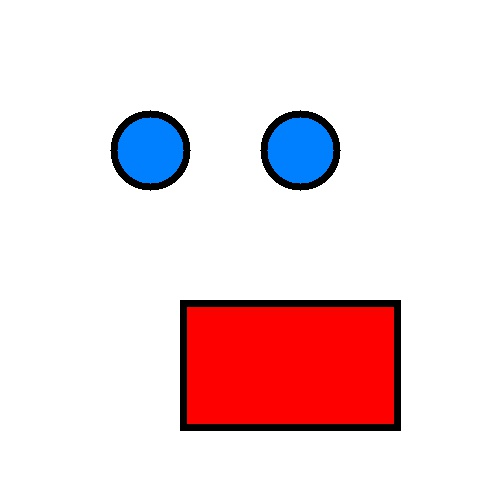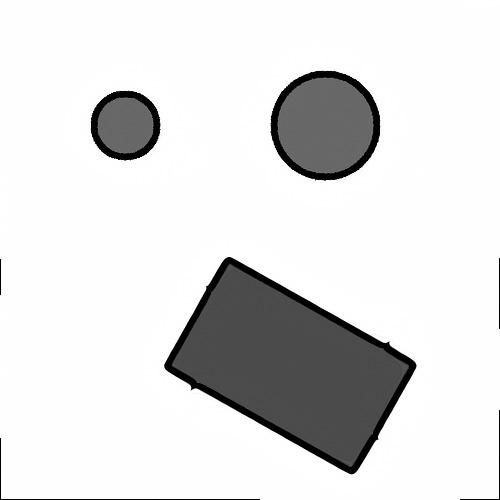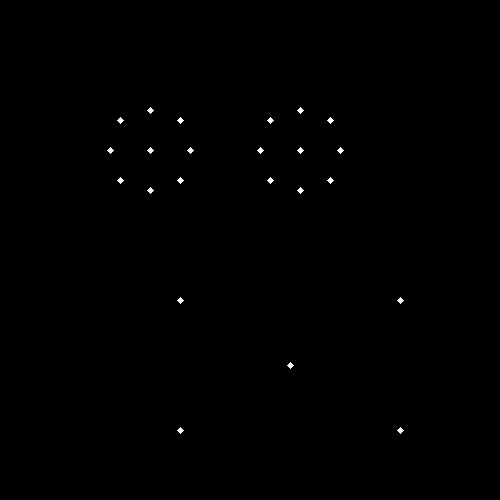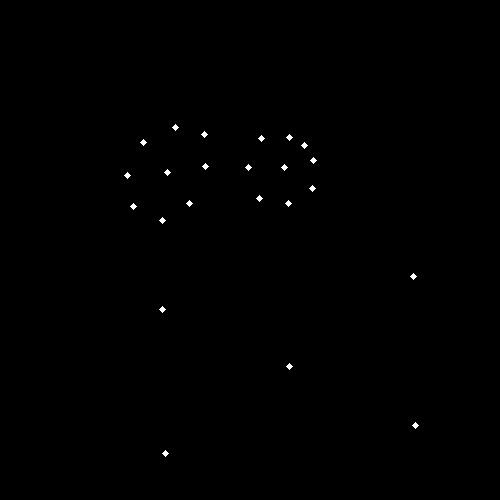# SimpleITK: Using TransformPoint() to Transform Mask Vertices

Hello,

I have a similar problem as the OP here but I’m attempting to use the `TransformPoint()` function from SimpleITK with a `DisplacementFieldTransform` instead of an affine transform.

### Problem

I have a moving image with a set of vertices that define a mask. After applying SimpleITK’s SymmetricForcesDemonsRegistration I have a transform to go from one image to another and I want to use the transform to find the new locations of mask vertices in the fixed image.Moving Image (Image with set mask vertices)Fixed Image (Image to find new location of mask vertices in)Moving image after applying SymmetricForcesDemonsRegistration

## Question:

Is there a way to use `TransformPoint()` to find the new vertices based on `DisplacementFieldTransform`?

It looks like the transformed vertices are the inverse of the original transformation but I am unsure how to rectify that.Incorrect mask vertices and centroids after applying `Transform.TransformPoint()`

### Attempts:

• I have tried OP’s original suggestion of computing the inverse transform with `GetInverse()` which throws an error saying that the inverse could not be created.
• I have also tried `GetInverseDisplacementField()` paired with `sitk.DisplacementFieldTransform` to generate the inverse with no luck either.
• I also generated an image with the vertices highlighted and then warped that image using the SimpleITK resampler to execute the transform. This successfully produced the right vertices however, it introduced other issues when I attempted to extract the vertices from the image. Using `TransformPoint()` would be the simplest and easiest if I can get it to work.

Any suggestions would be greatly appreciated.

Thanks.

Hello @bbikdash,

The output of the registration is a transformation which maps points from the fixed image to the moving image space.

If all you need is to map points from the current moving image to the fixed image, just switch their roles in the registration.

If you want to resample the current moving image onto the fixed image coordinate system and you want to map points from the moving image to the fixed image, you will need to estimate the inverse transformation to perform the latter. There are several options for estimating the inverse (different algorithms):

They all expect an image as input which you obtain from the transform using its `GetDisplacementField` method.

2 Likes

Of course. Switching the inputs of the registration filter would give me what I need.

Thank you, @zivy .